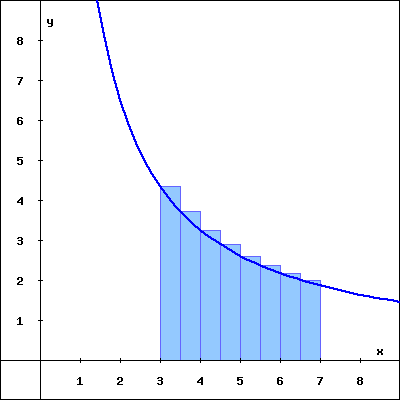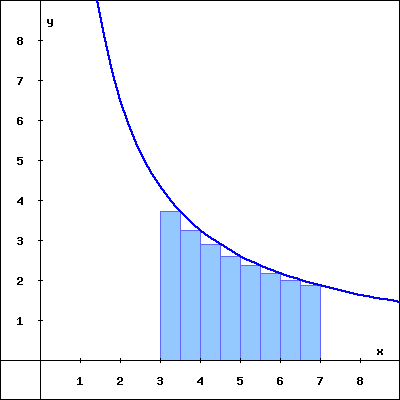The rectangles in the graph below illustrate a left endpoint Riemann sum for $\displaystyle f(x) = (13/x)$ on the interval $\lbrack 3, 7 \rbrack$.
The value of this left endpoint Riemann sum is , and this Riemann sum is the area of the region enclosed by $\displaystyle y = f(x)$, the x-axis, and the vertical lines x = 3 and x = 7.Left endpoint Riemann sum for $y = (13/x)$ on $\lbrack 3, 7 \rbrack$

The rectangles in the graph below illustrate a right endpoint Riemann sum for $\displaystyle f(x) = (13/x)$ on the interval $\lbrack 3, 7 \rbrack$.
The value of this right endpoint Riemann sum is , and this Riemann sum is the area of the region enclosed by $\displaystyle y = f(x)$, the x-axis, and the vertical lines x = 3 and x = 7.Right endpoint Riemann sum for $y = (13/x)$ on $\lbrack 3, 7 \rbrack$